## Mastering Important Concepts Tested by GMAT in Triangles - I

We invite all community contributors to share resources that are deemed to help students beat the GMAT. We've created individual threads to match the main topics, so please select the best fit for your content carefully.

### GMAT/MBA Expert

GMAT Instructor
Posts: 645
Joined: 26 Jul 2010
Location: US
Thanked: 527 times
Followed by:227 members

### Mastering Important Concepts Tested by GMAT in Triangles - I

by e-GMAT » Sun Dec 11, 2016 10:51 pm
Added the PDF of the article at the end of the post!Mastering Important Concepts Tested by GMAT in Triangles- I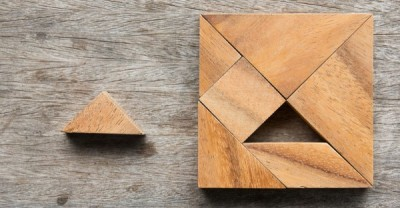1. OBJECTIVE OF THE ARTICLE

Geometry is a topic with which a lot of students are not comfortable and many test takers avoid solving geometry questions.

There are two main reasons for this:
• i) There are too many concepts and formulae to remember. Even though GMAT tests us on a very limited number of properties, but since these limited properties are not collated in a single place, it is difficult for a test taker to confidently say he knows all the concepts.

ii) And say, even if someone manages to find and learn all the concepts, most of them are not sure how to apply these concepts to deduce information from the given question and figure in the questions tested on the GMAT

• i) Consolidating the MOST important concepts needed in Triangles.

ii) Focusing on some GMAT-like and official questions and illustrating the application of the above concepts in these questions.

The article is broadly divided into three sections

1. Basic Properties of a triangle:

This section covers the following aspects:
• "¢ Finding if a set of given numbers could be the lengths of a triangle or not,
"¢ The range of values the side of a triangle can take given the values of the other two sides and
"¢ The properties of the angles of the triangle.

2. Tabular Representation:

Different types of triangles with their MOST important properties.

3. Special properties:

A few special properties that can be tested by GMAT.

Note: This post will cover just the first section!

So let's get started!

2. BASIC PROPERTIES OF A TRIANGLE

2.1 CONCEPT 1

"Sum of lengths of any two sides of a triangle > Length of the third side"

Suppose you are given three lengths a, b, and c, and asked to find out if we can form a triangle using these three lengths.

How are you going to approach this problem?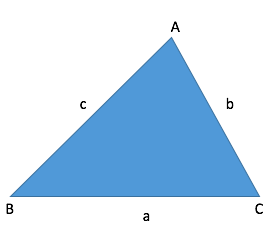Theory:

To determine if the three lengths will form a triangle or not.

We need to know the most basic property of a triangle:

Sum of lengths of any two sides of a triangle > Length of the third side

This means that:
• "¢ a + b > c
"¢ b + c > a
"¢ c + a > b
Please note that all the three inequalities should satisfy for the triangle to exist.

Let us understand this with the help of a very basic GMAT-like question :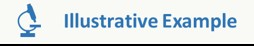Q. The three lengths 5, 3, and x are used to form a triangle. Which of the following can be the value of x?
• A. 6
B. 9
C. 10
D. 12
E. 14

Solution

Since 5, 3 and x form a triangle, they must satisfy the property -

Sum of lengths of any two sides of a triangle > Length of the third side

Therefore, we can write :

• I. 5+3 >x
x <8

II. 3 +x > 5
x > 2

III. 5 + x > 3
x > -2
So we can conclude that the range of x is: 2 < x < 8

Out of the given options, the only option that falls within this range is 6. Hence, Option A is our answer.

Therefore, now we know:
• "¢ How to find if the given lengths form a triangle or not
"¢ How to use the above property to find the range of values for an unknown side in a triangle.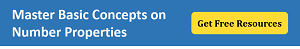2.2 CONCEPT 2

"In any triangle, the largest side is always opposite the largest angle and the smallest side is always opposite the smallest angle."

Suppose after finding out that the given lengths form a triangle, you want to find out how the length of the sides can help in finding a relation between the angles of the triangle.

To understand this, we need to know two more important properties -
• The sum of the interior angles of a triangle is constant and is equal to 180 degrees .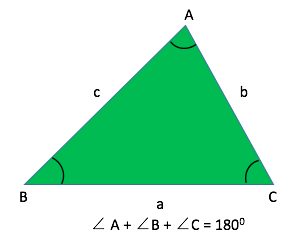Therefore,

A + B + C = 180

"¢ Please note that the above property holds true for all triangles irrespective of their size and shape.
(notice I am using capital A, B and C to denote the angles of the triangle and a, b and c for the lengths of the sides of the triangle)

And another property is:
• In any triangle, the largest side is always opposite the largest angle and the smallest side is always opposite the smallest angle.
Let me explain this a bit more in detail:

If ABC is a triangle, in which we know that the lengths AB, BC, and CA follow the relation AB>BC>AC, then we can conclude that, since AB is the greatest side the angle opposite to it i.e., angle ACB is the greatest angle.

And since BC > AC, angles opposite to them also hold the same relation, that means
angle BAC > angle ABC

Hence we can conclude that: âˆ ACB > âˆ BAC > âˆ ABC

Keep in mind that the converse is also true, i.e., if the relation between the angles is given, we can use that to find the relation between the sides.

Let us understand the application of this property through an example:In the triangle given below, all the points denoted are non-overlapping and the angles A, B, and C are distinct. Is AB>AC>BC?
• I. angle y1 = angle c1
II. angle BAC < angle ABC and neither angle is the greatest in the triangle ABC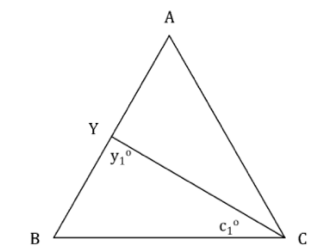Solution:

Approach

We need to find if the statement AB > AC > BC is true or not.

For this, it is enough to get a relation among angles A, B and C since the sides of a triangle follow the same relation as the angles opposite to them.

Analyse Statement 1

y1Â = c1

Therefore, in triangle BYC,

BY = BC

But AB = BY + YA
• "¢ AB > BC
However, we cannot infer any relationship between BC and AC (or AB and AC) from the given information.

Therefore, statement 1 is not sufficient to arrive at a unique answer.

Â Analyse Statement 2

Given angle BAC < angle ABC and neither angle is the greatest.

Also in the question statement it is mentioned that angles BAC, ABC, and ACB are distinct, therefore, angle ACB has to be the greatest angle.

Therefore, we can conclude, angle BAC < angle ABC < angle ACB

Thus, we can conclude that length BC < AC < AB

Hence, statement 2 is sufficient to arrive at a unique answer.

This brings us to end of the first section of the article. I hope it was useful and informative. After reading this you should be able to easily -

• 1. apply Concept 1, to identify if a triangle can be formed from the given three lengths.
2. apply Concept 1, to also find the range of the unknown side, if the other two lengths of the triangle are given.
3. apply Concept 2 in questions where the relation of angles and sides are required to solve the problem.

NOTE: This is the second article in our Geometry series. If you liked the above article, you can also go through the link given below. I am sure you will find it usefulCommon Mistakes in Geometry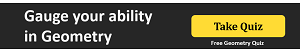Thanks,
Saquib
Quant Expert
e-GMAT

NOTE: This is the second article in our Geometry series. If you liked the above article, you can also go through the link given below. I am sure you will find it usefulAttachments
Triangle_Part1_BTG.pdf